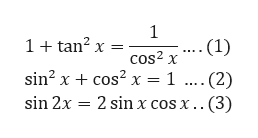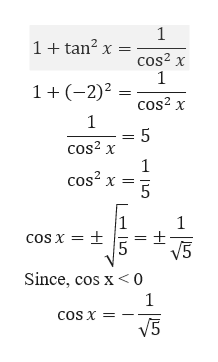Question
78 views

2x, given tan x= 2 and cos x is less than 0

check_circle

Step 1

Given tan x = 2 and cos x < 0.

Step 2

The Trigonometric identities to be used:help_outlineImage Transcriptionclose1+ tan? x = -(1) cos? x sin? x + cos? x = 1 .... (2) sin 2x = 2 sin x cos x.. (3) fullscreen
Step 3

Given tan x = 2. First fi...help_outlineImage Transcriptionclose1+ tan? x = cos? x 1+(-2)² cos? x cos? x cos? x = cos x = + V5 Since, cos x< 0 cos x = V5 COS X fullscreen

### Want to see the full answer?

See Solution

#### Want to see this answer and more?

Solutions are written by subject experts who are available 24/7. Questions are typically answered within 1 hour.*

See Solution
*Response times may vary by subject and question.
Tagged in
MathTrigonometry

### Trigonometric Ratios